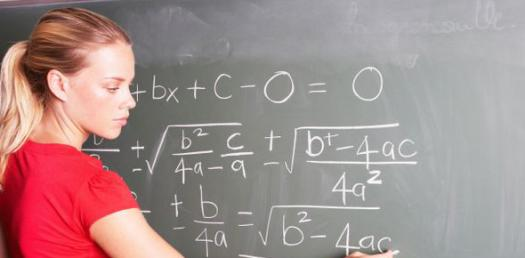# A Quiz About Quick Math

Approved & Edited by ProProfs Editorial Team
At ProProfs Quizzes, our dedicated in-house team of experts takes pride in their work. With a sharp eye for detail, they meticulously review each quiz. This ensures that every quiz, taken by over 100 million users, meets our standards of accuracy, clarity, and engagement.
| Written by Saycufwa909
S
Saycufwa909
Community Contributor
Total Contribution - 6 | Total attempts - 17,479
Questions: 18 | Attempts: 265SettingsMultiplication of numbers gets a bit harder when it comes to double digit numbers when compared to single digit numbers. The quick math quiz below is perfect for anyone who is trying to perfect their single digit multiplication skills. Give the one below a try and perfect your skills when it comes to multiplying numbers by 6. All the best!

• 1.

### 6*8=

• A.

48

• B.

12

• C.

68

A. 48
Explanation
The correct answer is 48 because when you multiply 6 by 8, you get the product of 48.

Rate this question:

• 2.

### 6*2=

• A.

8

• B.

62

• C.

12

C. 12
Explanation
The correct answer is 12 because when you multiply 6 by 2, you get the product of 12.

Rate this question:

• 3.

### 6*6=

• A.

66

• B.

36

• C.

12

B. 36
Explanation
The correct answer is 36 because when you multiply 6 by 6, you get the product of 36.

Rate this question:

• 4.

### 6*9=

• A.

37

• B.

26

• C.

54

C. 54
Explanation
The correct answer is 54 because when you multiply 6 by 9, you get the product of 54.

Rate this question:

• 5.

### 6*7=

• A.

47

• B.

42

• C.

48

B. 42
Explanation
The correct answer is 42 because when you multiply 6 by 7, you get the product of 42.

Rate this question:

• 6.

### 6*5=

• A.

30

• B.

35

• C.

25

A. 30
Explanation
The correct answer is 30 because when you multiply 6 by 5, you get the product of 30.

Rate this question:

• 7.

### 6*3=

• A.

24

• B.

81

• C.

18

C. 18
Explanation
The correct answer is 18 because when you multiply 6 by 3, you get the product of 18.

Rate this question:

• 8.

### 6*1=

• A.

12

• B.

6

• C.

78

B. 6
Explanation
The multiplication of 6 by 1 equals 6.

Rate this question:

• 9.

### 6*4=

• A.

24

• B.

42

• C.

12

A. 24
Explanation
The multiplication operation between 6 and 4 results in 24.

Rate this question:

• 10.

### 6*11=

• A.

65

• B.

67

• C.

66

C. 66
Explanation
The correct answer is 66 because when you multiply 6 by 11, you get the product of 66.

Rate this question:

• 11.

### 6*10=

• A.

60

• B.

4

• C.

71

A. 60
Explanation
The multiplication of 6 and 10 equals 60.

Rate this question:

• 12.

• A.

64

• B.

2

• C.

72

C. 72
• 13.

### 6*8=

• A.

43

• B.

48

• C.

81

B. 48
Explanation
The correct answer is 48 because when you multiply 6 by 8, you get the product of 48.

Rate this question:

• 14.

• A.

42

• B.

21

• C.

12

C. 12
• 15.

### 6*9=

• A.

45

• B.

54

• C.

66

B. 54
Explanation
Multiplying 6 by 9 gives the product of 54.

Rate this question:

• 16.

### 6*7=

• A.

42

• B.

69

• C.

65

A. 42
Explanation
The correct answer is 42 because when you multiply 6 by 7, you get the product of 42.

Rate this question:

• 17.

### 6*11=

• A.

55

• B.

77

• C.

66

C. 66
Explanation
The correct answer is 66 because when you multiply 6 by 11, you get the product of 66.

Rate this question:

• 18.

### 6*12=

• A.

72

• B.

27

• C.

34Back to top